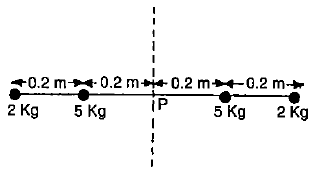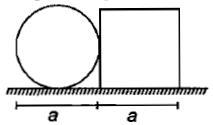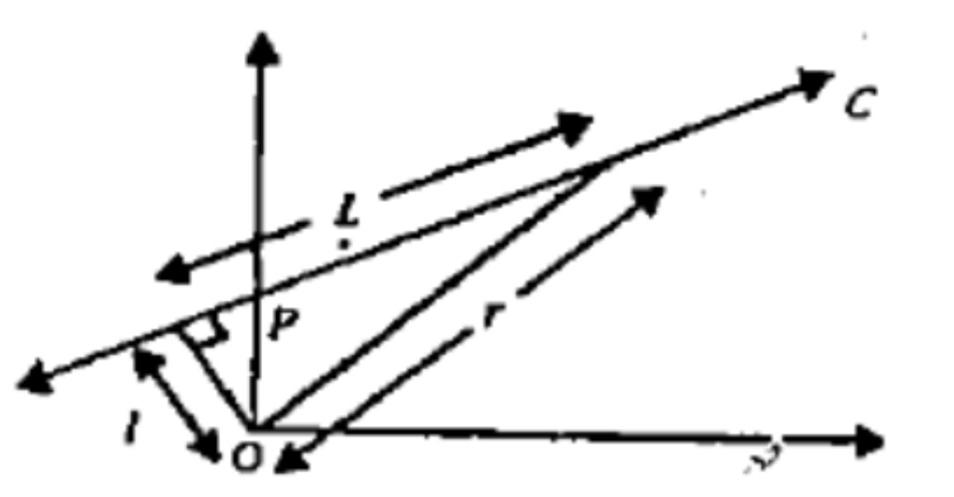A particle moves in a force field given by , where r is the unit vector along with the position vector $\stackrel{\to }{\mathrm{r}}$, then which is true?

1.  The torque acting on the particle is not zero

2.  The torque acting on the particle produces an angular acceleration in it

3.  The angular momentum of the particle is conserved

4.  The angular momentum of the particle increases.

Concept Questions :-

Angular momentum
High Yielding Test Series + Question Bank - NEET 2020

Difficulty Level:

The ratio of rotational and translatory kinetic energies of the sphere is

1.  2/9

2.  2/7

3.  2/5

4.  7/2

Concept Questions :-

Rolling motion
High Yielding Test Series + Question Bank - NEET 2020

Difficulty Level:

Two perfectly elastic particles A and B of equal mass traveling along the line joining them with velocities 15 m/s and 10 m/s. After the collision, their velocities will be

1.  10 m/s, 10 rn/s

2.  15 m/s, 15 m/s

3.  10 rn/s, 15 m/s

4.  15 m/s, 10 m/s

Concept Questions :-

Linear momentum
High Yielding Test Series + Question Bank - NEET 2020

Difficulty Level:

Four masses are fixed on a massless rod as shown in the figure. The moment of inertia about the axis P is about1.  2 kg-${\mathrm{meter}}^{2}$

2.  1 kg-${\mathrm{meter}}^{2}$

3.  0.5 kg-${\mathrm{meter}}^{2}$

4.  0.3 kg-${\mathrm{meter}}^{2}$

Concept Questions :-

Moment of inertia
High Yielding Test Series + Question Bank - NEET 2020

Difficulty Level:

A circular plate of diameter d is kept in contact with a square plate of an edge as shown in the figure. The density of the material and the thickness are the same everywhere. The center of mass of the composite system will be1.  inside the circular plate

2.  inside the square plate

3.  at the point of contact

4.  outside the system

Concept Questions :-

Center of mass
High Yielding Test Series + Question Bank - NEET 2020

Difficulty Level:

A small disc of radius 2 cm is cut from a disc of radius 6 cm. If the distance between their centres is 3.2 cm, what is the shift in the centre of mass of the disc?

1.  0.4 cm

2.  2.4 cm

3.  1.8 cm

4.  1.2 cm

Concept Questions :-

Center of mass
High Yielding Test Series + Question Bank - NEET 2020

Difficulty Level:

From a circular disc of radius R, a square is cut out with a radius as its diagonal.  The centre of mass of remainder is at a distance (from the centre)

(1) $\frac{R}{\left(4\mathrm{\pi }-2\right)}$             (2) $\frac{R}{2\mathrm{\pi }}$                (3) $\frac{R}{\left(\mathrm{\pi }-2\right)}$                     (4) $\frac{R}{\left(2\mathrm{\pi }-2\right)}$

High Yielding Test Series + Question Bank - NEET 2020

Difficulty Level:

A particle of mass m moves along line PC with velocity v as shown.  What is the angular momentum of the particle about P?(1) mvL                 (2) mvl                (3) mvr                  (4) zero

High Yielding Test Series + Question Bank - NEET 2020

Difficulty Level:

Two bodies with moment of inertia  $\left({I}_{1}>{I}_{2}\right)$ and have equal angular momenta.  If KE of rotation are , then

(1) ${E}_{1}={E}_{2}$                (2) ${E}_{1}>{E}_{2}$                (3)  ${E}_{1}<{E}_{2}$              (4) ${E}_{1}\ge {E}_{2}$

High Yielding Test Series + Question Bank - NEET 2020

Difficulty Level:

A mass m with velocity u strikes a wall normally and returns at the same speed. What is the magnitude of the change in momentum of the body when it returns

1.  4 mu

2.  mu

3.  2 mu

4.  0

Concept Questions :-

Linear momentum